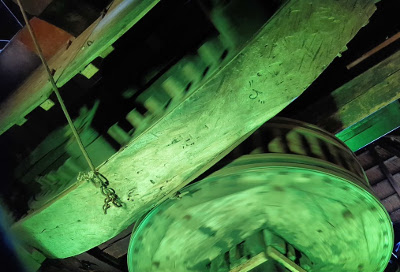## Classes and Class Creation

### Behind the scenes: Relationship between Class and typeIn this chapter of our tutorial, we will provide you with a deeper insight into the magic happening behind the scenes, when we are defining a class or creating an instance of a class. You may ask yourself: "Do I really have to learn theses additional details on object oriented programming in Python?" Most probably not, or you belong to the few people who design classes at a very advanced level.

First, we will concentrate on the relationship between type and class. When you have defined classes so far, you may have asked yourself, what is happening "behind the lines". We have already seen, that applying "type" to an object returns the class of which the object is an instance of:

x = [4, 5, 9]
y = "Hello"
print(type(x), type(y))

The code above returned the following:
<class 'list'> <class 'str'>


If you apply type on the name of a class itself, you get the class "type" returned.

print(type(list), type(str))

The above Python code returned the following:
<class 'type'> <class 'type'>


This is similar to applying type on type(x) and type(y):

x = [4, 5, 9]
y = "Hello"
print(type(x), type(y))
print(type(type(x)), type(type(y)))

This gets us the following result:
<class 'list'> <class 'str'>
<class 'type'> <class 'type'>


A user-defined class (or the class "object") is an instance of the class "type". So, we can see, that classes are created from type. In Python3 there is no difference between "classes" and "types". They are in most cases used as synonyms.

The fact that classes are instances of a class "type" allows us to program metaclasses. We can create classes, which inherit from the class "type". So, a metaclass is a subclass of the class "type".

Instead of only one argument, type can be called with three parameters:

type(classname, superclasses, attributes_dict)

If type is called with three arguments, it will return a new type object. This provides us with a dynamic form of the class statement.

• "classname" is a string defining the class name and becomes the name attribute;
• "superclasses" is a list or tuple with the superclasses of our class. This list or tuple will become the bases attribute;
• the attributes_dict is a dictionary, functioning as the namespace of our class. It contains the definitions for the class body and it becomes the dict attribute.

Let's have a look at a simple class definition:

class A:
pass
x = A()
print(type(x))

After having executed the Python code above we received the following result:
<class '__main__.A'>


We can use "type" for the previous class defintion as well:

A = type("A", (), {})
x = A()
print(type(x))

The previous Python code returned the following result:
<class '__main__.A'>

Generally speaking, this means, that we can define a class A with
type(classname, superclasses, attributedict)
When we call "type", the call method of type is called. The call method runs two other methods: new and init:
type.__new__(typeclass, classname, superclasses, attributedict)
type.__init__(cls, classname, superclasses, attributedict)


The new method creates and returns the new class object, and after this the init method initializes the newly created object.

class Robot:
counter = 0
def __init__(self, name):
self.name = name
def sayHello(self):
return "Hi, I am " + self.name
def Rob_init(self, name):
self.name = name
Robot2 = type("Robot2",
(),
{"counter":0,
"__init__": Rob_init,
"sayHello": lambda self: "Hi, I am " + self.name})
x = Robot2("Marvin")
print(x.name)
print(x.sayHello())
y = Robot("Marvin")
print(y.name)
print(y.sayHello())
print(x.__dict__)
print(y.__dict__)

The previous Python code returned the following result:
Marvin
Hi, I am Marvin
Marvin
Hi, I am Marvin
{'name': 'Marvin'}
{'name': 'Marvin'}


The class definitions for Robot and Robot2 are syntactically completely different, but they implement logically the same class.

What Python actually does in the first example, i.e. the "usual way" of defining classes, is the following: Python processes the complete class statement from class Robot to collect the methods and attributes of Robot to add them to the attributes_dict of the type call. So, Python will call type in a similar or the same way than we did in Robot2.